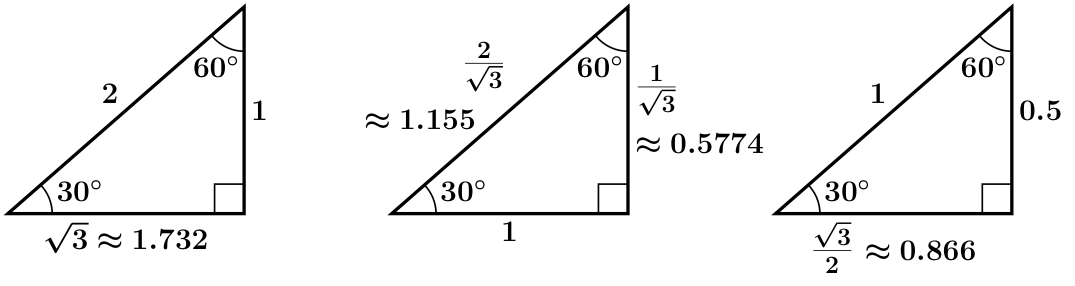# GEOM 2 | Lesson 2 | Explore & Watch

Now that you understand the relationship among the 3 sides in special right triangles, it is time to look at the ratio among 2 sides in right triangles.

Look at the following problem:

The school needs to build new ramps around the campus that all have a ratio of the vertical rise to horizontal run to be 1:12. This is the regulation for a ramp to be within ADA guidelines. We are going to compare two of the ramps.

1) One ramp needs to have a vertical rise of 3 feet.

2) The other ramp needs to have a vertical rise of 5 feet.

Calculate the horizontal run of each ramp. Then calculate the length of each ramp.

Compare the ramps to each other by looking at the ratio of each vertical rise, each horizontal run, and each length of the ramp. What do you notice about the ratios? What can you say about the 2 triangles that form the ramps?

Next, compare the rise and run of the following three special right triangles.Calculate the ratio of the rise to the run of each triangle. What do you notice?

What is another way to define “rise” over “run”?

Compare the “rise” and “run” of each ramp.

Watch the following video on tangent:

Define tangent, and identify that the angle of reference for all of those tangent values.

Next, calculate tangent of all three 60 degree angles. What can you say about tangent values for angles in similar triangles?

Next compare the values of tan (30) and tan (60).

Repeat this process for tangent for the two 45 degree angles in the following triangles.How would you find a missing angle in a right triangle if you only know the 90 degree angle, but know 2 of the sides?

Inverse Trig Ratios (Soh-Cah-Toa)

Use inverse tangent to solve the following problem:Find the measure of $$\angle{X}$$ and $$\angle{Y}$$.